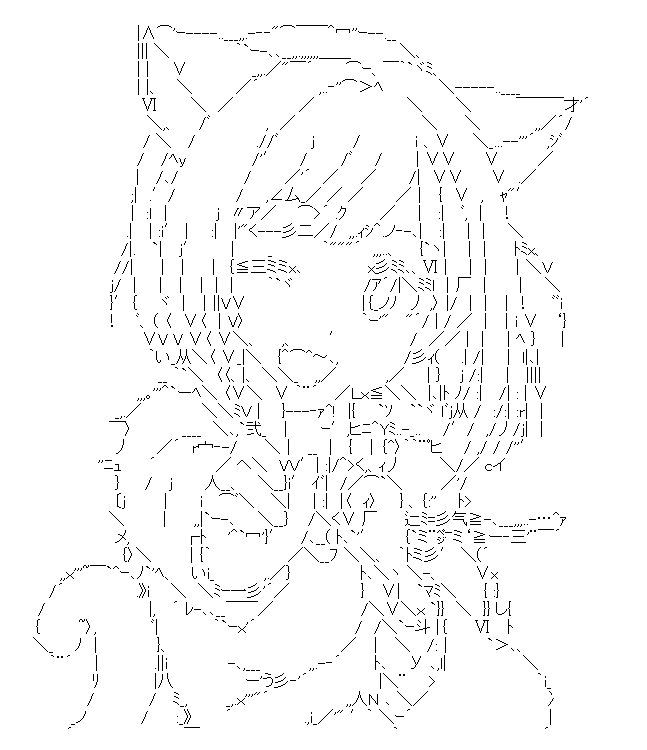# DeepAA

This is convolutional neural networks generating ASCII art. This repository is under construction.

This work is accepted by NIPS 2017 Workshop, Machine Learning for Creativity and Design The paper: ASCII Art Synthesis with Convolutional Networks## Requirements

• TensorFlow (1.3.0)
• Keras (2.0.8)
• NumPy (1.13.3)
• Pillow (4.2.1)
• Pandas (0.18.0)
• Scikit-learn (0.19.0)
• h5py (2.7.1)
• model's weight (download it from here and place it in dir `model`.)
• training data (additional, download it from here, extract it and place the extracted directory in dir `data`.) )

## How to use

please change the line 15 of `output.py`

``````image_path = 'sample images/original images/21 original.png' # put the path of the image that you convert.
``````

into the path of image file that you use. You should use a grayscale line image.

then run `output.py`. converted images will be output at `output/`.

You can select light model by change the line 13, 14 of `output.py` into

``````model_path = "model/model_light.json"
weight_path = "model/weight_light.hdf5"
``````